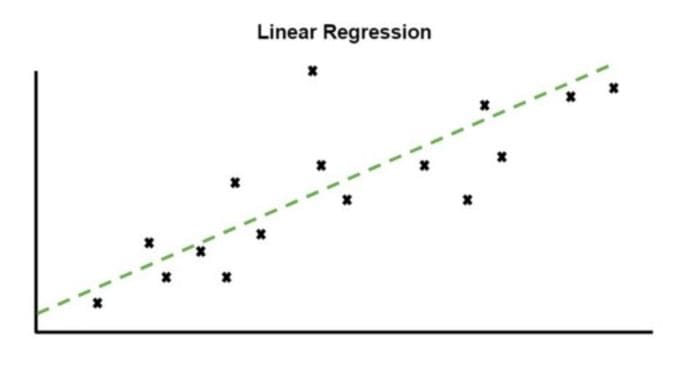By Zachary Warnes, Data ScientistPhoto by Cesar Carlevarino Aragon on Unsplash

This post is meant for new and or aspiring data scientists trying to decide what model to use for a problem.

This post will not be going over data wrangling. Which hopefully, you know, is the majority of the work a data scientist does. I’m assuming you have some data ready, and you want to see how you can make some predictions.

## Simple Models

There are many models to choose from with seemingly endless variants.

There are usually only slight alterations needed to change a regression model into a classification model and vice versa. Luckily this work has already been done for you with the standard python supervised learning packages. So you only need to select what option you want.

There are a lot of models to choose from:

• Decision trees
• Support vector machines (SVM)
• Naive Bayes
• K-Nearest Neighbors
• Neural Networks
• Random Forests

The list goes on and on, but consider starting with one of two.

## Linear regression & Logistic regressionPhoto by iMattSmart on Unsplash

Yes, fancy models like xgboost, BERT, and GPT-3 exist, but start with these two.

Note: logistic regression has an unfortunate name. The model is used for classification, but the name persists due to historical reasons.

I would suggest changing the name to something straightforward like linear classification to remove this confusion. But, I don’t have that kind of leverage in the industry yet.

## Linear Regression

``````from sklearn.linear_model import LinearRegression
import numpy as npX = np.array([[2, 3], [5, 6], [8,9], [10, 11]])
y = np.dot(X, np.array([1, 2])) + 1
reg = LinearRegression().fit(X, y)
reg.score(X, y)``````

## Logistic Regression

``````from sklearn.linear_model import LogisticRegression
clf = LogisticRegression(solver="liblinear", random_state=10).fit(X, y)
clf.score(X,y)``````

## Why These Models?

Busting out some deep learning model and spending hundreds on AWS fees to get only a slight accuracy bump is not worth it.

These two models have been studied for decades and are some of the most well-understood models in machine learning.

They are easily interpretable. Both models are linear, so their inputs translate to their output in a way that you could calculate by hand.

Even if you are an experienced data scientist, you should know the performance of these models on your problem, mainly because they are so effortless to implement and test.

I’ve been guilty of this. I’ve dived straight in and built complex models before. Thinking the xgboost model I’m using is overall superior, so it should be my starting model. Only to find out that a linear regression model performs with a few percentage points. And linear regression was used because it is more straightforward and more interpretable.

There is an element of ego at play here.Photo by Sebastian Herrmann on Unsplash

You may want to show that you understand these complex models and how to use them. But they’re just sometimes not practical to set up, train and maintain. Just because a model can be used does not mean that model should be used.

Don’t waste your time. Something good enough and gets used is always better than something intricate, but no one uses or understands.

So hopefully, now you start simple and start with one of these models.

## The First Question

Is my problem a classification problem or a regression problem?

### Is your problem a regression problem?

Are you trying to predict a continuous output?Linear Regression (Photo by Author)

The price of something like a house, a product, or a stock? Regression.

How long will something last, like flight duration, manufacturing time, or time a user spends on your blog? Regression.

Save the performance here. If it is already good enough for your problem, then go with it. Otherwise, now you can start experimenting with other models.

### Is your problem a classification problem?

Are you trying to predict a binary output or multiple unique and discrete outputs?Logistic Regression (Photo by Author)

Are you trying to determine if someone will buy something from your store or win a game? Classification.

Does a yes or no answer the question you have? Classification.

Start with logistic regression, make a scatter plot of your data or a subset of it and color the classes. Maybe there is already a clear pattern.

Again, evaluate the model and use this as your baseline if you still need to improve your performance. But start here.

## Conclusion

Likely, those who have read through this will find themselves in a similar situation, selecting what model to use. And then deciding your problem is perfect for this new model from a paper you read. As a result, spending hours fine-tuning this complex model only to have a more straightforward model win in the end.

Not necessarily by performance, but because precisely because they are simple and easy to interpret.

Save yourself some time and energy. Just start with linear regression and logistic regression.

Bio: Zachary Warnes is a Data Scientist at Pacmed, and an individual who continually seeks out new challenges. Years ago, realizing that tackling new problems and overcoming obstacles is the fastest way to learn and develop new skills, Zachary has sought to continually place myself in new situations in order to benefit from facing each new challenge.

Original. Reposted with permission.

Related: